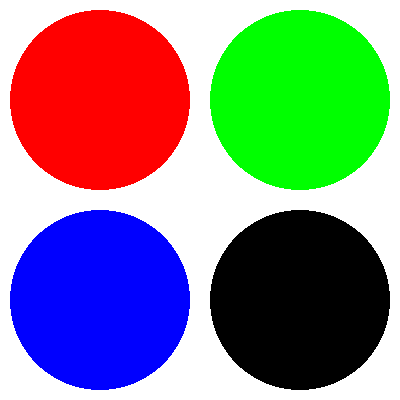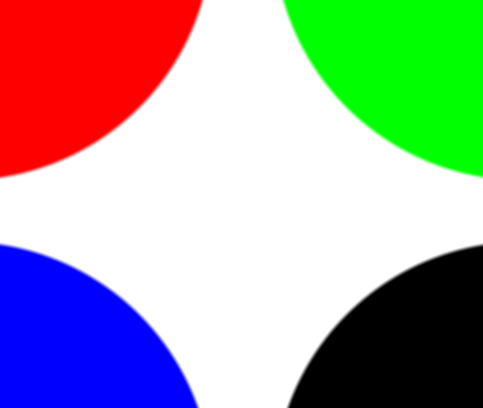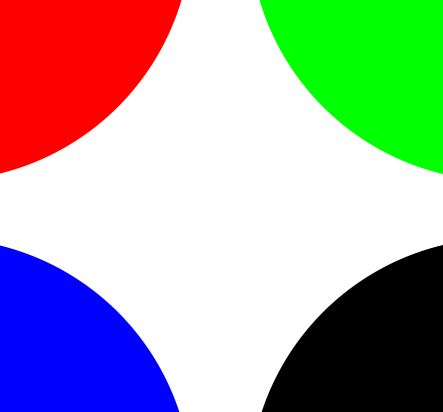# Drawing antialiased circles in OpenGL

In this post we will look at the `fwidth` function in GLSL, and how it can be used to draw circles (or other 2D shapes) with resolution independent antialiasing. We will be drawing the circles in the fragment shader, using a threshold on the distance from the center of a quad that fills the entire OpenGL viewport (modeled by two triangles). The simple way to draw four circles on this quad is as shown in the following fragment shader:

``````#version 330

in vec2 fPosition;
out vec4 fColor;

void main() {
vec4 colors = vec4[](
vec4(1.0, 0.0, 0.0, 1.0),
vec4(0.0, 1.0, 0.0, 1.0),
vec4(0.0, 0.0, 1.0, 1.0),
vec4(0.0, 0.0, 0.0, 1.0)
);
fColor = vec4(1.0);

for(int row = 0; row < 2; row++) {
for(int col = 0; col < 2; col++) {
float dist = distance(fPosition, vec2(-0.50 + col, 0.50 - row));
float alpha = step(0.45, dist);
fColor = mix(colors[row*2+col], fColor, alpha);
}
}
}
``````

This produces the following image:As you can see these circles have some not so pretty jagged edges. This happens because we have a sharp cutoff between pixels that are completely within the required distance from the center, and pixels that are only partially within the required distance from the center.

We can make the circles look better by using a more gradual transition function from an `alpha` of 0 to 1. This is what the `smoothstep(a, b, x)` function is for. If `x <= a` or `x >= b` it produces 0 and 1 respectively, like `step`. However if `a < x < b` the result is a smooth interpolation between 0 and 1 based on the position of `x` in the interval `[a, b]`.

Using `smoothstep` we can get a better value for `alpha`:

``````#version 330

in vec2 fPosition;
out vec4 fColor;

void main() {
// [...]

for(int row = 0; row < 2; row++) {
for(int col = 0; col < 2; col++) {
float dist = distance(fPosition, vec2(-0.50 + col, 0.50 - row));
float delta = 0.1;
float alpha = smoothstep(0.45-delta, 0.45, dist);
fColor = mix(colors[row*2+col], fColor, alpha);
}
}
}
``````

We get the following image:Unfortunately it turns out our value of `0.1` for `delta` is too high. With some trial and error, we find that a value of `0.01` produces a much better balance between blurriness and jaggedness:However, watch what happens when we increase the resolution of this image and look at the center portion:The edges are blurry again! This happens because our value for `delta` is not resolution independent. No matter how small you choose delta, as long as it is bigger than 0 you will always be able to increase the resolution to a point where the edges become blurry, as `delta` stays constant.

In order to solve this you could pass the current resolution to the shader as uniforms and somehow calculate a suitable `delta` from that, but there is a much easier and better way, which is to use `fwidth`. Simply said, `fwidth(x)` will calculate a measure of the difference between `x` in the current fragment shader and the `x` values in the neighbouring fragments. As such it can be used to approximate the width of the current fragment in terms of `x`.

If we use `fwidth(dist)` we know how much the distance from the center varies from one fragment to the next, and we can decide to have a smooth transition on the edge of the circle that is approximately one fragment wide:

``````#version 330

in vec2 fPosition;
out vec4 fColor;

void main() {
// [...]

for(int row = 0; row < 2; row++) {
for(int col = 0; col < 2; col++) {
float dist = distance(fPosition, vec2(-0.50 + col, 0.50 - row));
float delta = fwidth(dist);
float alpha = smoothstep(0.45-delta, 0.45, dist);
fColor = mix(colors[row*2+col], fColor, alpha);
}
}
}
``````

Now we get nice smooth circles without having to guess a good value for `delta`:Additionally, we have gained resolution independence. When we increase the resolution, the edges are still antialiased, but sharply defined instead of blurry:The same technique can be used to draw all sorts of antialiased shapes such as polygons, letters, lines, etc.